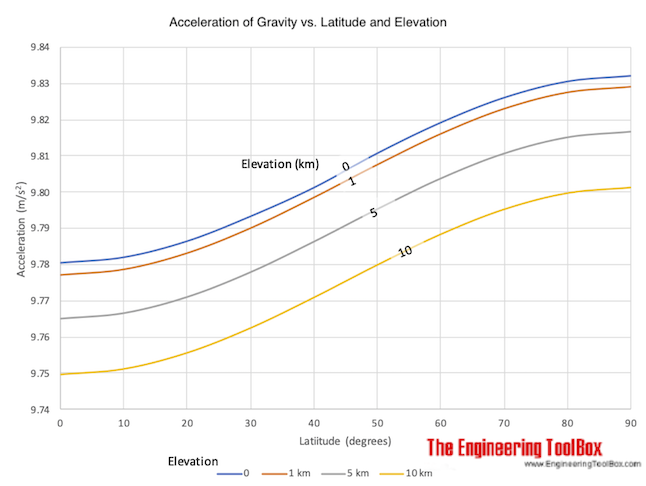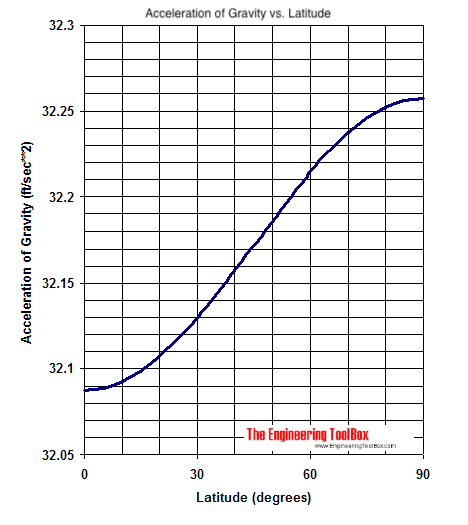Engineering ToolBox - Resources, Tools and Basic Information for Engineering and Design of Technical Applications!

# Acceleration of Gravity vs. Latitude and Elevation

## Acceleration of gravity due to latitude and elevation above sea level.

Acceleration of gravity at sea level and elevation related to north-south position on earth (degrees latitude):

### Acceleration of Gravity vs. Latitude and Elevation (m/s2)### Acceleration of Gravity vs. Latitude (ft/s2)### Example - Time for Falling Object to Hit the Ground on the Pole or on the Equator

The distance traveled after some time by a free falling object can be expressed as:

s = 1/2 ag t2

where

s = distance (m, ft)

ag = acceleration of gravity (m/s2, ft/s2

t = time (s)

The acceleration of gravity is stronger at the poles than at equator and the equation above can be modified to

t = (2 s / ag)1/2

The time for an object at level 1 m to hit the ground on the pole can be calculated as:

t = (2 (1 m) / (9.832 m/s2))1/2

= 0.4510 s

The time for an object at level 1 m to hit the ground on the equator can be calculated as:

t = (2 (1 m) / (9.78 m/s2))1/2

= 0.4522 s

### Example - The Weight of a Large Man in Canada vs. Venezuela

The acceleration of gravity in Canada at latitude 60 degrees is approximately 9.818 m/s2 and the acceleration of gravity in Venezuela at latitude 5 degrees is approximately 9.782 m/s2.

The weight - or gravity force - of a large man with mass 100 kg in Canada can be calculated as

Fg = (100 kg) (9.818 m/s2)

= 982 N

The weight the man in Venezuela can be calculated as

Fg = (100 kg) (9.782 m/s2)

= 978 N

## Related Topics

• Miscellaneous - Engineering related topics like Beaufort Wind Scale, CE-marking, drawing standards and more.

## Engineering ToolBox - SketchUp Extension - Online 3D modeling!

Add standard and customized parametric components - like flange beams, lumbers, piping, stairs and more - to your Sketchup model with the Engineering ToolBox - SketchUp Extension - enabled for use with the amazing, fun and free SketchUp Make and SketchUp Pro .Add the Engineering ToolBox extension to your SketchUp from the SketchUp Pro Sketchup Extension Warehouse!

Translate

## Privacy

We don't collect information from our users. Only emails and answers are saved in our archive. Cookies are only used in the browser to improve user experience.

Some of our calculators and applications let you save application data to your local computer. These applications will - due to browser restrictions - send data between your browser and our server. We don't save this data.

## Citation

• Engineering ToolBox, (2009). Acceleration of Gravity vs. Latitude and Elevation. [online] Available at: https://www.engineeringtoolbox.com/acceleration-gravity-latitude-d_1554.html [Accessed Day Mo. Year].

Modify access date.

. .

#### Scientific Online Calculator3 30